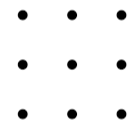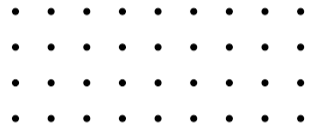# MaxagonDraw some polygons by joining the dots on a $3$ by $3$ grid.

You can find an interactive environment and dotty paper to print off on the dotty grids resource page.What is the greatest number of sides that your polygon could have?

What about on a $3$ by $4$ grid, or a $3$ by $5$ grid?

What about on a $3$ by $n$ grid?

Can you explain the pattern by which the 'number of sides' increases?

Next, explore some polygons on grids that are $4$ dots high.What is the maximum number of sides a polygon could have on a $4$ by $n$ grid?

Can you explain how you know?

What is the maximum number of sides a polygon could have on a $6$ by $6$ grid?

And on a $6$ by $n$ grid?

With thanks to Don Steward, whose ideas formed the basis of this problem.

### The biggest number of sides a

The biggest number of sides a polygon could have in a 3 by 3 grid is 5 sides

### I think you can do better...

Keep trying - you may find you can draw a polygon with more than 5 sides on a 3 by 3 grid. You are allowed reflex angles.

### The biggest number of sides

I think the biggest number of sides on a 3 by 3 grid would be 8 sides

### The biggest number of sides

Can you describe how to draw it?

I found that
3 by 3 = 8 sides,
3 by 4 = 10 sides,
and...

they keep going up by 2 sides each time.

Then I labelled 3 by 3 as size 1, 3 by 4 as size 2, etc.

This led to the formula (where size is n):
maximum number of sides = 2n + 6

If n = 1:
2 x 1 + 6 = 8
If n = 2:
2 x 2 + 6 = 10

I think you might want to check those, it can be easy to accidentally count a side twice.

Can you describe what your shapes look like? It might be useful to use co-ordinates of some sort.
For example, you could use (0,0) for the bottom left dot and (2, 0) for the bottom right dot.
Or you could use letters for the columns and numbers for the rows, or whatever you find easiest.
Just make sure to explain what you're using.

### Solution and formula

I did the 3x3 and got 6 but then thought there could be more sides.
After some trial and error I found a 7-sided shape by modifying it.

Then on the 3x4 I got an 8 followed by one with 10 sides.

This led me to a formula:

$xy-2$ which is multiplying the number of dots on the two sides together before subtracting 2.

I did this for the 4x4 and got, 4 x 4 - 2 = 14 sides. Then I found some shapes to fit it.

I did the same for 4x5 and got 18 sides.
Then for 4x6 and I got a 22. It looked like a rocket!

### Solution and formula

That's an interesting formula, but are you sure that it works for every grid?

To try to make as many sides as possible, we want to use every dot (once, otherwise we won't have a polygon) and we want to change direction each time (so that we make a new side every time we go to a new dot).

I tried this with the 4 by 4 grid and I reached every dot and changed direction every time, so I know I ended up with the maximum number of sides.

For some grids you might not be able to reach every dot or change direction every time. For which grids does this happen?

We may need different formulas for different grids...